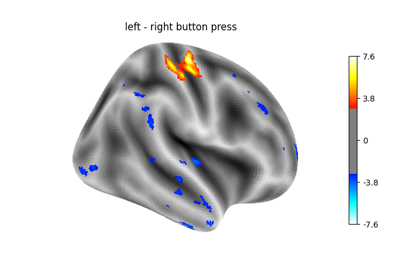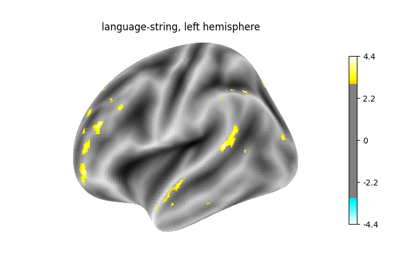Note

This page is a reference documentation. It only explains the class signature, and not how to use it. Please refer to the user guide for the big picture.

3.9.1. nistats.contrasts.Contrast¶

class nistats.contrasts.Contrast(effect, variance, dim=None, dof=10000000000.0, contrast_type='t', tiny=1e-50, dofmax=10000000000.0)

The contrast class handles the estimation of statistical contrasts on a given model: student (t) or Fisher (F). The important feature is that it supports addition, thus opening the possibility of fixed-effects models.

The current implementation is meant to be simple, and could be enhanced in the future on the computational side (high-dimensional F constrasts may lead to memory breakage).

__init__(self, effect, variance, dim=None, dof=10000000000.0, contrast_type='t', tiny=1e-50, dofmax=10000000000.0)
Parameters
effectarray of shape (contrast_dim, n_voxels)

the effects related to the contrast

variancearray of shape (n_voxels)

the associated variance estimate

dim: int or None,

the dimension of the contrast

dofscalar

the degrees of freedom of the residuals

contrast_type: {‘t’, ‘F’}

specification of the contrast type

effect_size(self)

effect_variance(self)

p_value(self, baseline=0.0)

Return a parametric estimate of the p-value associated with the null hypothesis: (H0) ‘contrast equals baseline’

Parameters
baselinefloat, optional

baseline value for the test statistic

Returns
p_values1-d array, shape=(n_voxels,)

p-values, one per voxel

stat(self, baseline=0.0)

Return the decision statistic associated with the test of the null hypothesis: (H0) ‘contrast equals baseline’

Parameters
baselinefloat, optional

Baseline value for the test statistic

Returns
stat: 1-d array, shape=(n_voxels,)

statistical values, one per voxel

z_score(self, baseline=0.0)

Return a parametric estimation of the z-score associated with the null hypothesis: (H0) ‘contrast equals baseline’

Parameters
baseline: float, optional,

Baseline value for the test statistic

Returns
z_score: 1-d array, shape=(n_voxels,)

statistical values, one per voxel

3.9.1.1. Examples using nistats.contrasts.Contrast¶